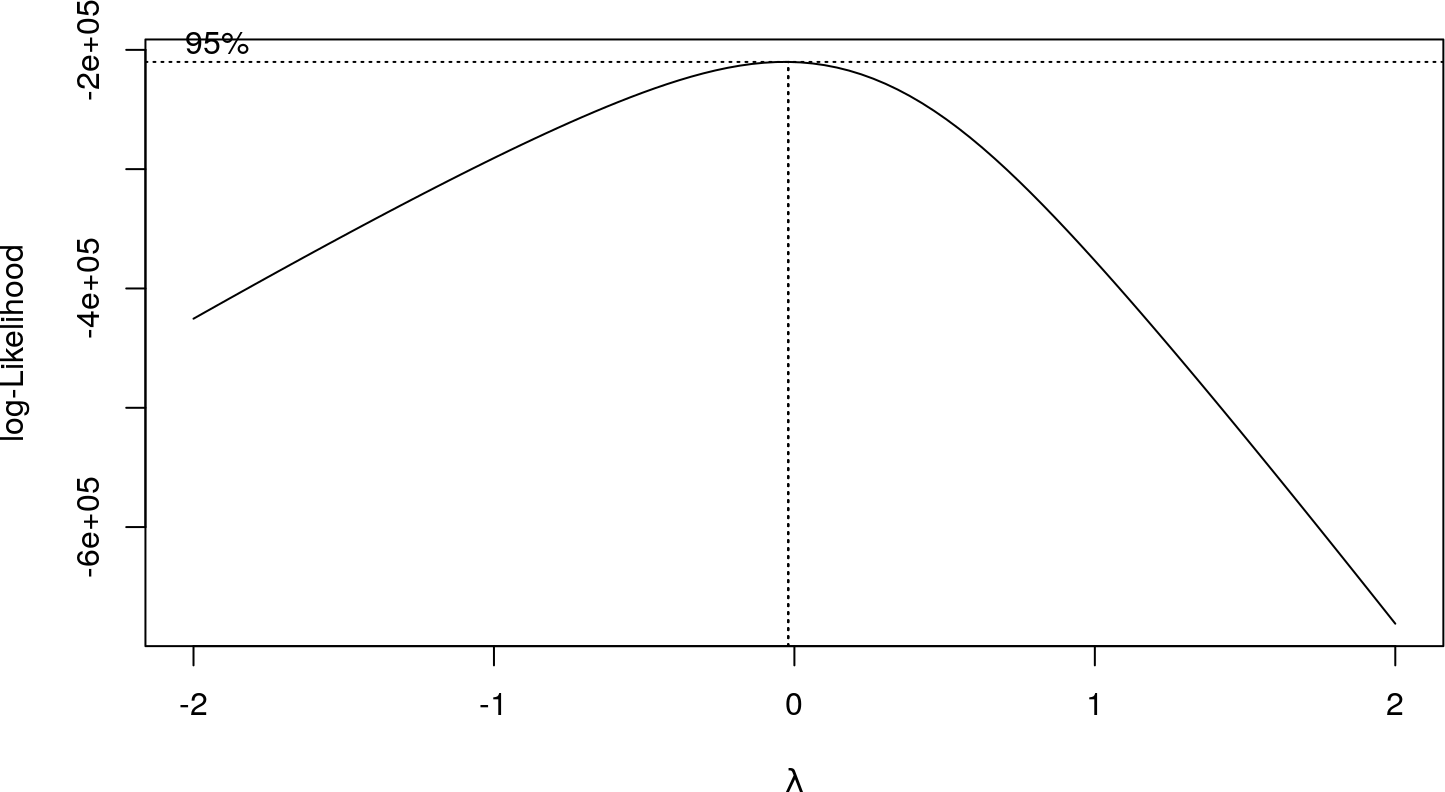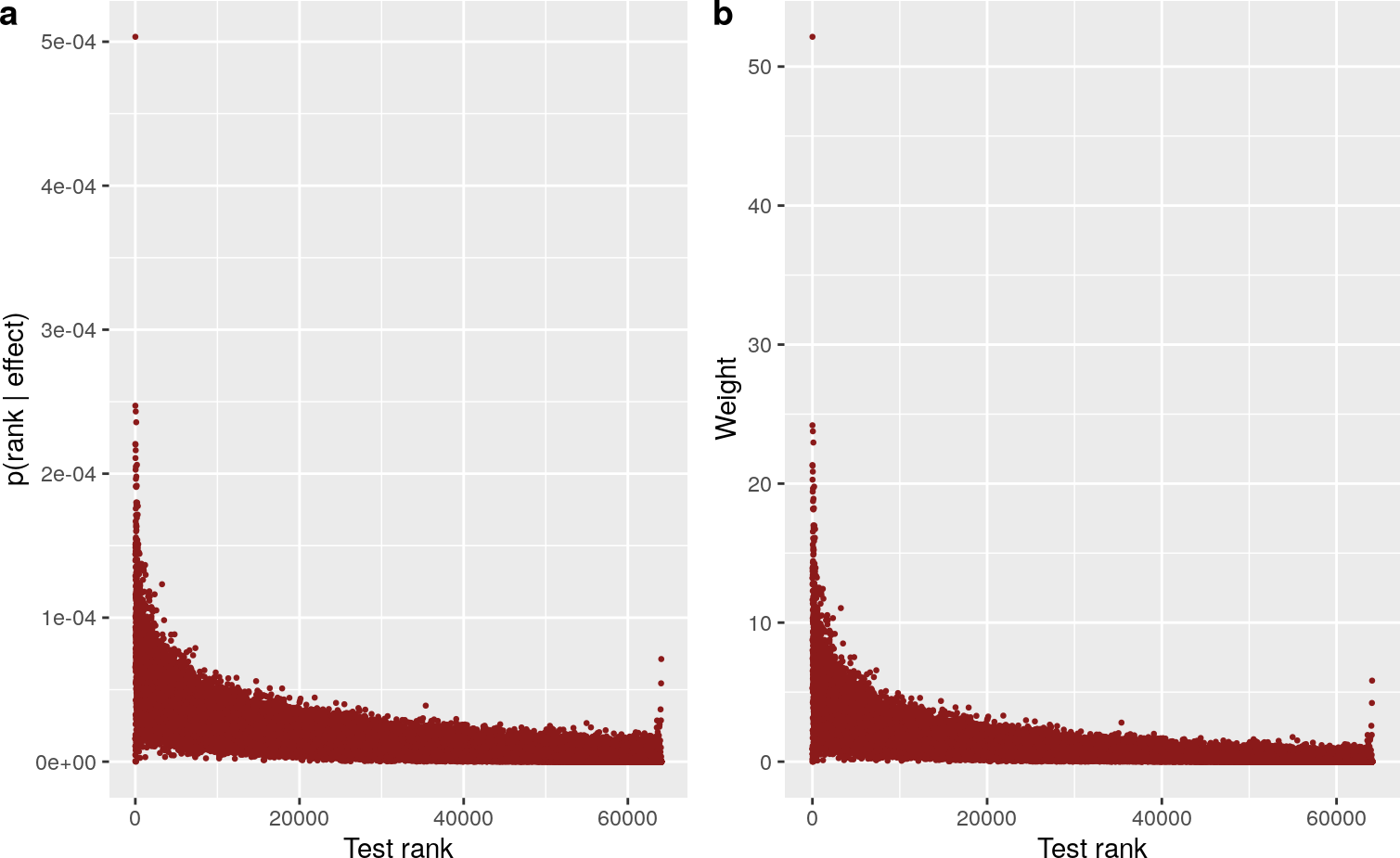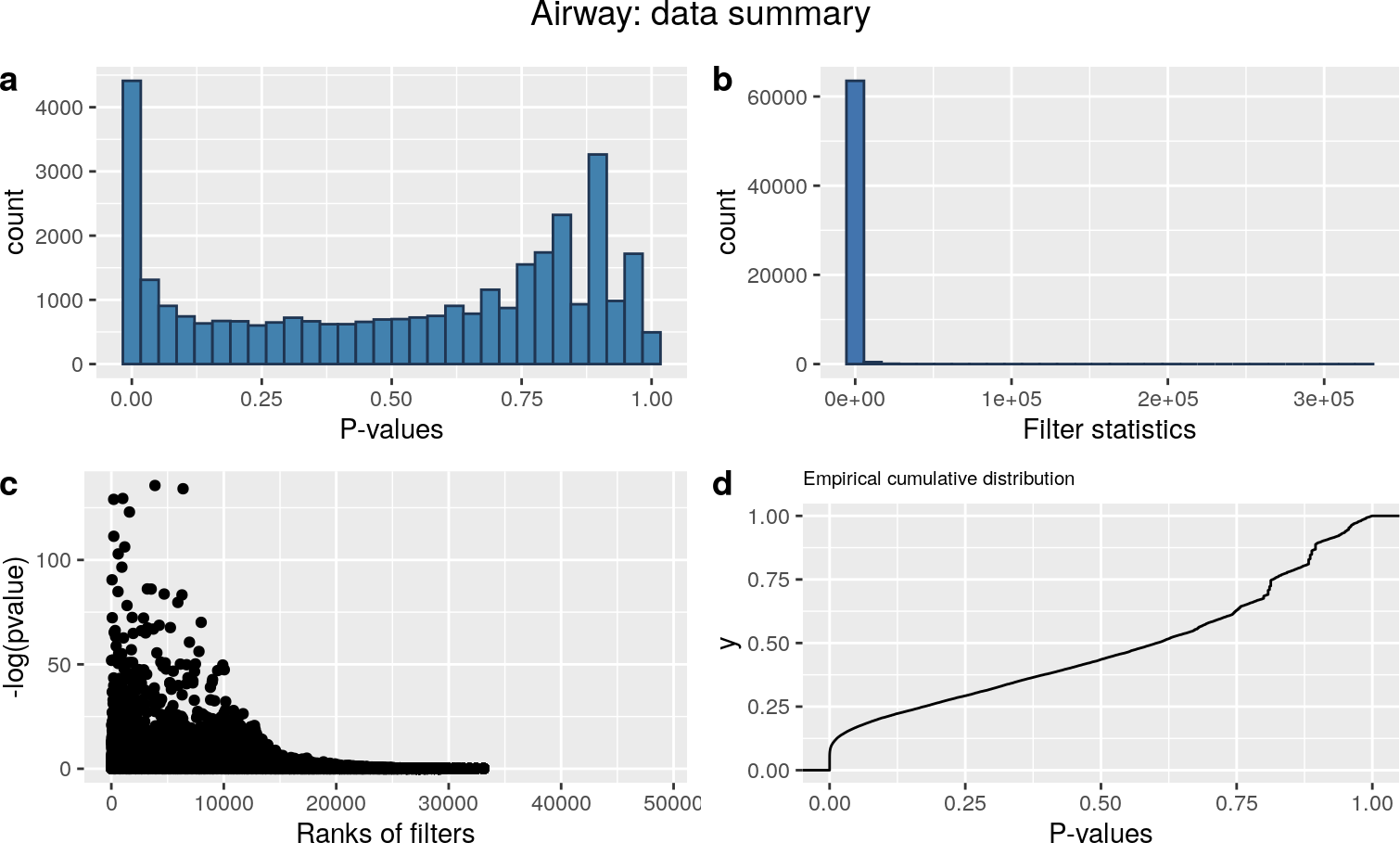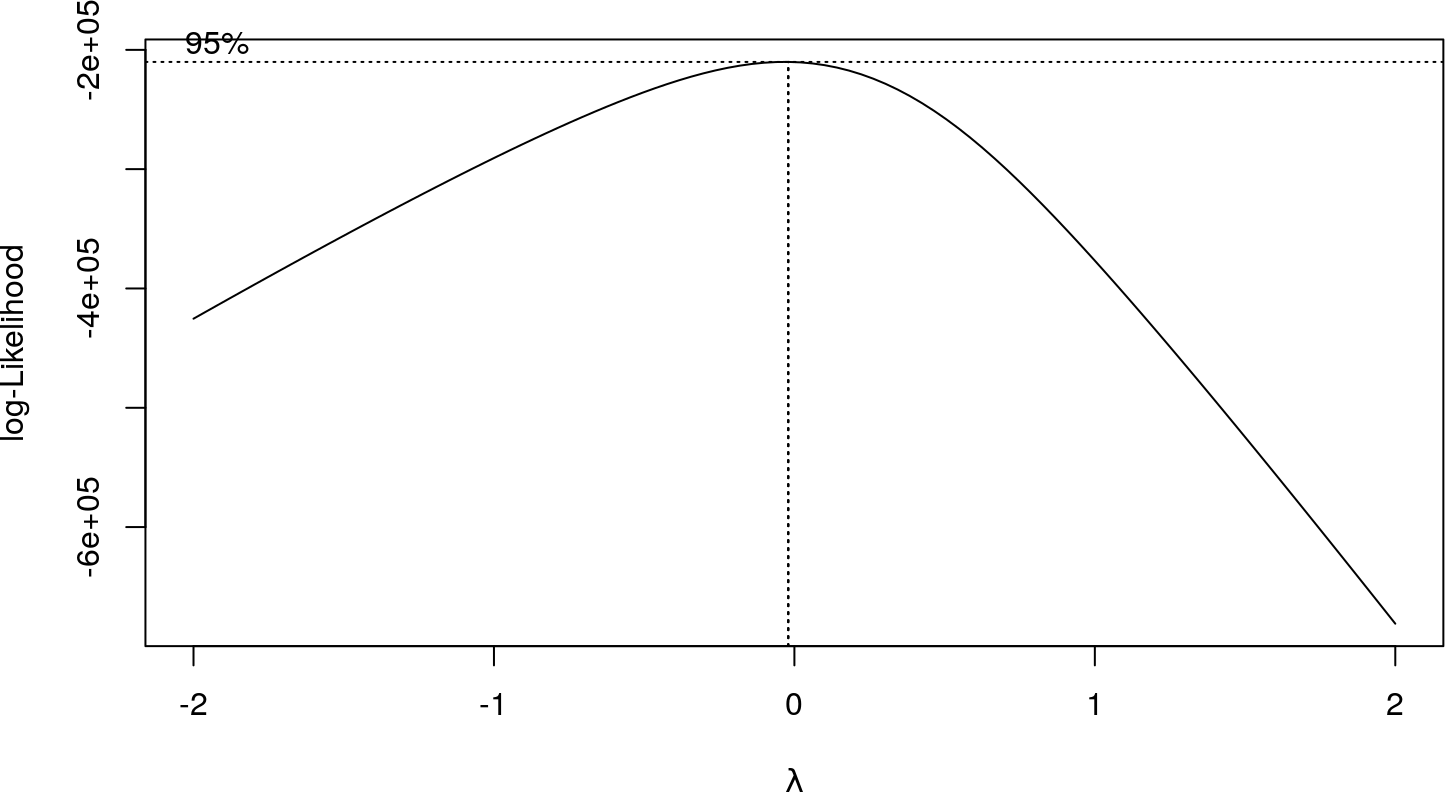# 1 Introduction

High throughput data is very common in modern science. The main property of these data is high-dimensionality; that is, the number of features is larger than the number of observations. There are many ways to study this kind of data, and multiple hypothesis testing is one of them. In a multiple hypothesis test, generally a list of p-values $$(p_i)$$ are calculated, one for each hypothesis $$(H_i)$$ corresponding to one feature; the p-values are then compared with one or more predefined fixed or random thresholds to obtain the number of significant features. The key goal is to control the Family Wise Error Rate ($$FWER$$) or False Discovery Rate ($$FDR$$) while maximizing the power of the tests.

Although multiple hypotheses provide platform to test many features simultaneously, it often requires high compensation for doing so (Stephens 2016). To overcome from this shortcoming, (Benjamini, Hochberg, and Kling 1997) shows a way of using the rank of the p-values frequently termed as adjusted p-values. However, this method solely depends on the p-values and, therefore, provides only sub optimal power of the tests. An alternative approach is p-value weighting in which external information is used in terms of weight and the actual p-value is redefined by incorporating the weight, which is usually accomplished dividing the original p-values by the corresponding weights and creating new p-values called weighted p-values, i.e., $$p_i^{w_i} = \frac{p_i}{w_i}; i = 1, ...., m$$, where $$p_i^{w_i}$$ and $$w_i$$ refers to the weighted p-value and the corresponding weight. This external information frequently referred as the filter statistics or the covariates $$(y_i)$$. The requirements of the method are that the covariates are assumed to be independent under the null hypothesis but informative for the power (Bourgon, Gentleman, and Huber 2010). In addition, the weights must be non-negative, and the mean of the weights must be equal to $$1$$.

Generally, covariates provide different prior probabilities of the null hypotheses being true; therefore, a judiciously chosen covariate can significantly improve the power of the test while maintaining the error rate below the threshold. Such covariates are frequently available from various studies and data sets (Ignatiadis et al. 2016). In this vignette, we discussed an application of a newly proposed method of the p-value weighting called Optimal Pvalue Weighting (OPW), which will soon appear in the journal. In the article, we showed how to compute an optimal weight of the p-values without estimating the effect sizes of the tests. We showed that the weights can be computed by applying a probabilistic relationship of the ranking of the tests and the effect sizes. In regards to $$OPW$$, we developed an $$R$$ package named OPWeight, and we will discuss the application procedures of the functions of the package. To apply the function, the essential inputs are:

1. a vector of p-values
2. a vector of filter statistics, where each value corresponds to a p-value

and the auxiliary inputs are

1. the probabilities of the rank of the test statistics given the mean effect size
2. mean of the filter and/or test effect sizes
3. a significance level of $$\alpha$$ at which $$FWER$$ or $$FDR$$ will be controlled

The proposed $$OPW$$ method uses covariates to compute the probability of the ranks of the test statistics being higher than any other tests given the mean effect size, $$p(r_i \mid \varepsilon_i)$$, then compute the weights from the probability corresponding to the p-values. As an example, we will discuss an RNA-seq differential gene expression data called airway from the $$R$$ library airway. This data set is also used in the $$IHW$$ package vignettes (Ignatiadis et al. 2016).

# 2 Airway RNA-seq data example

We fist preprocess the airway RNA-Seq data set using DESeq2 (Love, Huber, and Anders 2014) to obtain the p-values, test statistics, and the covariates (filter statistics).

# install OPWeight package
if (!requireNamespace("BiocManager", quietly=TRUE))
install.packages("BiocManager")

library("OPWeight")
library("ggplot2")
library("airway")
library("DESeq2")
library(cowplot)
library(tibble)
library(qvalue)
library(MASS)

Process RNA-seq data

data("airway", package = "airway")
dds <- DESeqDataSet(se = airway, design = ~ cell + dex)
dds <- DESeq(dds)
de_res_air <- results(dds)

The output is a DESeqResults object containing the following columns for each gene in which each gene corresponds to a hypothesis test:

colnames(de_res_air)
##  "baseMean"       "log2FoldChange" "lfcSE"          "stat"
##  "pvalue"         "padj"
dim(de_res_air)
##  64102     6

From the above columns, we will consider baseMean as the covariate. In the DESeq2 paper, it has argued that the covariate baseMean and the test statistics stat are approximately independent under the null hypothesis, which fulfills the requirements for the baseMean to be the filter statistics.

First we will load the proposed package OPWeight and then show the steps to conduct $$OPW$$ tests:

filters = de_res_air$baseMean + .0001 # add a small constant to make all values positive set.seed(123) # one should use more nrep to obtain more acurate results opw_results <- opw(pvalue = de_res_air$pvalue, filter = filters, nrep = 1000,
alpha = .1, tail = 2, effectType = "continuous", method = "BH")Figure 1: The above plot shows the estimated $$\lambda$$ of the box-cox transformation and the corresponding $$log-likelihood.$$

The executed function $$opw()$$ returns a list of objects:

names(opw_results)
##  "totalTests"      "nullProp"        "ranksProb"       "weight"
##  "rejections"      "rejections_list"

For example, the estimated proportion of the true null hypothesis:

opw_results$nullProp ##  0.8987421 The number of rejected null hypothesis: opw_results$rejections
##  5101

The plots of the probabilities, $$P(r_i \mid \varepsilon_i)$$, and the corresponding weights, $$w_i$$:

m = opw_results$totalTests testRanks = 1:m probs = opw_results$ranksProb
weights = opw_results$weight dat = tibble(testRanks, probs, weights) p_prob = ggplot(dat, aes(x = testRanks, y = probs)) + geom_point(size = .5, col="firebrick4") + labs(x = "Test rank" , y = "p(rank | effect)") p_weight = ggplot(dat, aes(x = testRanks, y = weights)) + geom_point(size = .5, col="firebrick4")+ labs(x = "Test rank" , y = "Weight") plot_grid(p_prob, p_weight, labels = c("a", "b"))Figure 2: Rank probability (a) and Weight (b) versus Test rank If one wants to test $$H_0: \varepsilon_i=0$$ vs. $$H_0: \varepsilon_i > 0$$, i.e., the effect sizes follow continuous distribution, then one should use effectType = "continuous". Similarly, if one wants to test $$H_0: \varepsilon_i=0$$ vs. $$H_0: \varepsilon_i=\varepsilon$$, i.e., effect sizes are binary; $$0$$ under the null model and a fixed value $$\varepsilon$$ under the alternative model, one should use effectType = "binary". $$opw()$$ function can provide results based on $$FDR$$ or $$FWER$$, and the default method is Benjamini-Hochberg (Benjamini, Hochberg, and Kling 1997) $$FDR$$ method. The main goal of the function is to compute the probabilities of the ranks from the p-values and the filter statistics, consequently the weights. Although weights and ranksProb are optional, $$opw$$ has the options so that one can compute the probabilities and the weights externally if necessary (see Data analysis section). Other parameters that we did not use in the $$opw()$$ function can be discussed here. Internally, the function compute the ranksprob and consequently the weights, then uses the p-values to make conclusions about hypotheses. Therefore, if ranksprob is given then mean_filterEffect are redundant, and should not be provided to the funciton. Although ranksprob is not required to the function, One can compute ranksprob by using the function prob_rank_givenEffect. The function $$opw()$$ internally compute mean_filterEffect and mean_testEffect from a simple linear regression with box-cox transformation between the test and filter statistics, where the filters are regressed on the test statistics. Then the estimated mean_filterEffect and mean_testEffect are used to obtian the ranksprob and the weights. Thus, in order to apply the function properly, it is crucial to understand the uses of the parameters mean_filterEffect and mean_testEffect. Note that, filters need to be positive to apply $$box-cox$$ from the R library MASS If mean_filterEffect and mean_testEffect are not provided then the test statistics computed from the p-values will be used to obtain the relationship between the filter statistics and the test statistics to compute the mean effects. If one of the mean effects are not given, then the missing mean effect will be computed internally. There are many ways to obtain the mean of the test effects, mean_testEffect, and the corresponding value of the filter effects, mean_filterEffect; however, in the proposed $$R$$ function $$opw()$$, we used a simple linear regression with box-cox transformation. Sometimes the box-cox transformation may not be the optimal choice or one wants to use a different model to obtain the relationship. In that situation, one can acquire the relationship between the filter and test statistics externally then provide ranksprob or mean_filterEffect and mean_testEffect to perform the proposed optimal p-value weighting. In the following section, we will show the details analysis procedure. # 3 Data analysis Before applying the methods, perform a pre-screening analysis of the data set. Consider the above data set. First make some plots based on the filter statistics, and the p-values. Data <- tibble(pval=de_res_air$pvalue, filter=de_res_air$baseMean) hist_pval <- ggplot(Data, aes(x = Data$pval)) +
geom_histogram(colour = "#1F3552", fill = "#4281AE")+
labs(x = "P-values")

hist_filter <- ggplot(Data, aes(x = Data$filter)) + geom_histogram( colour = "#1F3552", fill = "#4274AE")+ labs(x = "Filter statistics") pval_filter <- ggplot(Data, aes(x = rank(-Data$filter), y = -log10(pval))) +
geom_point()+
labs(x = "Ranks of filters", y = "-log(pvalue)")

p_ecdf <- ggplot(Data, aes(x = pval)) +
stat_ecdf(geom = "step")+
labs(x = "P-values", title="Empirical cumulative distribution")+
theme(plot.title = element_text(size = rel(.7)))

p <- plot_grid(hist_pval, hist_filter, pval_filter, p_ecdf,
labels = c("a", "b", "c", "d"), ncol = 2)
## stat_bin() using bins = 30. Pick better value with binwidth.
## Warning: Removed 30633 rows containing non-finite values (stat_bin).
## stat_bin() using bins = 30. Pick better value with binwidth.
## Warning: Removed 30633 rows containing missing values (geom_point).
## Warning: Removed 30633 rows containing non-finite values (stat_ecdf).
# now add the title
title <- ggdraw() + draw_label("Airway: data summary")
plot_grid(title, p, ncol = 1, rel_heights=c(0.1, 1))Figure 3: (a) Distribution of the p-values, (b) distribution of the filter statistics, (c) relationship between the p-values and the rank of the filter statistics, and (d) empirical cumulative distribution of the p-values.

From the pre-analysis plots, we see the distribution of the filter statistics is rightly skewed; therefore, to perform a linear regression the filter statistics need to be transformed. In $$opw()$$ function, we used box-cox transformation. However, one can perform another transformation, such as $$log(filter)$$, and fit a simple linear regression model, or apply other methods to obtain the relationship. For example, generalized linear model. Although the transformed distribution may not be still suitable, this may not be a severe problem as long as the model fit the center of the data well, because the proposed method only requires the center of the distribution.

We also observe from the plot that there is a weak relationship between the test statistics and the filter statistics; however, the ranked-filter statistics shows a potential relationship with the p-values, i.e., low p-values are enriched at the higher filter statistics. This relationship might be informative for the p-value-weighting because we are more interested in the ranking of the filter statistics. Enrichment of the low p-values at higher filter statistics also indicates that the filter statistics is correlated with the power under the alternative model. Furthermore, the bimodal distribution of the p-values indicates two-tailed test criteria are necessary because p-values close to $$1$$ be the cases that are significant in the opposite direction. We also observed the empirical cumulative distribution of the p-values. The empirical cumulative distribution shows that the curve is almost linear for the high p-values, which reveals the lesser importance of the higher p-values, and the size of the low p-values is very small.

We first fit a linear regression with box-cox transformation to obtain the estimated mean_filterEffect and mean_testEffect in the following:

# initial stage--------
pvals = de_res_air$pvalue tests = qnorm(pvals/2, lower.tail = FALSE) filters = de_res_air$baseMean + .0001    # to ensure filters are postive for the box-cox

# formulate a data set-------------
Data = tibble(pvals, filters)
OD <- Data[order(Data$filters, decreasing=TRUE), ] Ordered.pvalue <- OD$pvals

# estimate the true null and alternative test sizes------
m = length(Data$pvals); m ##  64102 nullProp = qvalue(Data$pvals, pi0.method="bootstrap")$pi0; nullProp ##  0.8987421 m0 = ceiling(nullProp*m); m0 ##  57612 m1 = m - m0; m1 ##  6490 # fit box-cox regression #-------------------------------- bc <- boxcox(filters ~ tests)lambda <- bc$x[which.max(bc$y)]; lambda ##  -0.02020202 model <- lm(filters^lambda ~ tests) # etimated test efects of the true altrnatives------------ test_effect = if(m1 == 0) {0 } else {sort(tests, decreasing = TRUE)[1:m1]} # two-tailed test # for the continuous effects etimated mean effects mean_testEffect = mean(test_effect, na.rm = TRUE) mean_testEffect ##  3.792828 mean_filterEffect = model$coef[] + model$coef[]*mean_testEffect mean_filterEffect ##  0.9045375 In order to obtain the mean effects, we compute the predicted value of the filter statistics corresponding to the mean or median of the test statistics. The mean or median value of the test statistics is then used as the estimated mean_testEffect and the corresponding predicted value of the filter statistics is used as the estimated mean_filterEffect. Now compute the probabilities of the ranks of the filter statistics given the mean effect size computed above. Note that, for the ranks probability et and ey are the same, because mean effects of the filter statistics is only a single value. set.seed(123) prob_cont <- sapply(1:m, prob_rank_givenEffect, et = mean_filterEffect, ey = mean_filterEffect, nrep = 1000, m0 = m0, m1 = m1) Then applying the probabilities to compute the weights # delInterval can be smaller to otain the better results wgt <- weight_continuous(alpha = .1, et = mean_testEffect, m = m, delInterval = .01, ranksProb = prob_cont) Finally, obtain the total number and the list of the significant tests alpha = .1 padj <- p.adjust(Ordered.pvalue/wgt, method = "BH") rejections_list = OD[which((padj <= alpha) == TRUE), ] n_rejections = dim(rejections_list) n_rejections ##  5100 or apply $$opw$$ function to compute the final results opw_results2 <- opw(pvalue = pvals, filter = filters, weight = wgt, effectType = "continuous", alpha = .1, method = "BH") ## comparing pvalues with thresholds opw_results2$rejections
##  5100

The above procedures are performed by the $$opw()$$ function internally by default; however, if one wants to try a different approach such as fitting a generalized linear model instead of the box-cox transformation, then one can apply a different model and follow the similar procedures to compute the parameters mean_filterEffect and mean_testEffect, or weight or ranksProb, then apply the results in $$opw()$$ function. Sometime it is necessary to perfom parallel computing; especially if the number of the hypothesis tests are large (e.g. billions). In his context, following the data analysis procedure could be convenient instead of using the $$opw()$$ function directly. To make the parallel computing faster, one may need to split the weight_continuous function. That is, use weight_by_delta function outside of the weight_continuous function and thence compute the weihgts.

# 4 Other functions

In the package OPweight, there are other useful functions: 1) prob_rank_givenEffect, 2) weight_binary, 3) weight_continuous, 4) weight_by_delta, 5) prob_rank_givenEffect_approx, 6) prob_rank_givenEffect_exact, and 7) prob_rank_givenEffect_simu. First three functions are used inside the main function $$opw()$$, and the fourth is used inside the second and the third; however, the remaining three functions are provided if anyone wants to see the behavior of the probability of the rank of the test statistic, $$p(r_i \mid \varepsilon_i)$$. In the original, article we proposed the probability method and showed an exact mathematical formula then verify the formula by simulations. The exact method requires intensive computation; therefore, we proposed an approximation. By applying the later three functions one can easily observe that all the methods perform similarly. However, for a large number of tests the simulation and the exact approach are computationally very slow; therefore, the approximation method is a better option. In fact, we actually need $$p(r_i \mid E(\varepsilon_i))$$, which is obtained by the function prob_rank_givenEffect. For the details see Hasan and Schliekelman, (2017) and the corresponding supplementry materials.